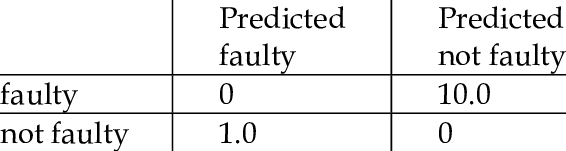# Impliment a Cost Matrix in Pytorch

Hey guys,

I’m kinda new to pytorch/ML in general and haven’t tried writing my own loss function before, I’m looking at Custom loss functions - #9 by ptrblck and it doesn’t seem too difficult. My specific question is to do with implementing a cost matrix which is what I think How to make cost function out of confusion matrix? is trying to do but it seems confusing.

Specifically I’m training a EfficientNet on the ISIC 2019 dataset and wanted to ask if there is any specific standard way for doing this in pytorch?

For those of you who don’t know a confusion matrix is something looks like this:Using this matrix I would multiply the loss by a factor of 10 if my model predicted ‘not faulty’ when it was in fact ‘faulty’, and multiply the loss by only a factor of 1 if it predicted something that was ‘not faulty’ as ‘faulty’ (and multiply by 0 if it got it right). My cost matrix won’t look like this exactly, as mine is on skin lesions and ISIC has 8 different unbalanced classes of skin cancer, and some classifications are more dangerous than others, which is why it’s important that my model learns which incorrect predictions are more costly.

Any help would be appreciated

Hi, did you find a way to solve this? I have a similar problem. Thanks!

Hey, sorry bout that, Forgot I made this post (and then subsequently my password). The way I’m implementing my cost matrix is not by building it into the loss function but instead by using the method defined in Bishops book “Pattern Recognition And Machine Learning”

Bishop gives us this formula to implement a cost matrix:This implementation effectively balances a softmax output of a neural network with the cost associated with misclassification.

e.g. lets say I have some probability distribution (0.35, 0.65) that is the softmaxed output from my network, so in this case we’re predicting 35% faulty and 65% not faulty. However, using Bishops sum I would get the Expected cost values of ((0.35 * 0 + 0.35 * 1), (10 * 0.65 + 0.65 * 0)) = (0.35, 6.5).

From these two expected cost values, 0.35 and 6.5, we choose the one that gives us the lowest expected cost, in this cause we predict faulty.

My explanation is likely rather poor, I believe Bishop talks about this in chapter 1.52 if you want to check that out.

1 Like